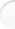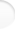Structure of Magic and Semi-Magic Squares, Methods and Tools for Enumeration by Francis GaspalouI give here some guidelines about a program of enumeration of:
- magic or semi-magic squares of order 5,
- pandiagonal squares of order 7,
- squares having specific properties, like bordered squares of order 5,
-...

 1. Algorithm

1.1 Principle

The algorithm is the same, whatever the order and the programming language (Basic, Pascal, C...). This algorithm is very simple. See in Appendix 2 the listing of an enumeration program (called by some authors "backtracking program") in Basic for order 3. This program is not optimized and has only a didactic interest.

You use imbricate loops (as many loops as parameters).

In each loop (that is to say for each parameter X), you use a "for... to...next" instruction (or equivalent) and a boolean variable T(X) which takes 2 status values, for example:
T(X)=0 means the value X is available,
T(X)=1 means the value X is not available.
You make tests for the parameter X and for the induced parameters (3 tests: value in the variation range, free value and induced parameters all different). If all the tests are successful, you occupy this value and the induced parameters, and you go on to the next parameter. If the tests are negative, you increment the parameter X.

At the end of each loop, when a solution has been found, you make free the value X and the induced values.

The number of cases to inspect increases very rapidly with the order n. You can have an upper limit of this number with this little calculus:
- for the first parameter, there are theoretically n2 cases to examine,
- for the second parameter, there are n2 - 1 cases to examine,
- etc.
Therefore, for N parameters, there are n2 * (n2- 1) * ... * (n2- N +1) cases to examine.
As N=(n-1)²-2 for magic squares, the last number is n2- (n-1)² + 3 = 2n + 2.
For n=3, you find 9 * 8 = 72 cases to examine
For n=4, you find 16 * 15 * ... * 10 = 57 657 600 cases to examine.
In fact, these numbers can be improved when you consider the real program and the induced parameters (one parameter can induce 2 cells or more in the square).

You see the interest of a reduced program (with the group G).

You see also the interest of any method that reduces the number of cases to examine. You can calculate for most parameters a lower boundary-stone and an upper boundary-stone (cf. improved program in Appendix 2, § 2). But if you reduce the range of variation of a given parameter, you have to balance with the necessary time to calculate these new lower and upper boundary-stones.

When searching the orthogonal squares to a given lower-case letter square, you reduce also the number of cases to examine.

Note that for some applications, it is better to use coordinates of cells with a couple (i,j) for the row i and the column j, instead of the notation A1, A2, ..., B1, B2,..., etc.

1.3 Other method

Instead to have one run with all the parameters with their full range of variation, it is often better to consider the place of the number 1 in the square and to have as many runs as necessary (in mathematical language, the number of runs is the number of partitions of the set of cells).

Ex: for enumeration of magic squares of order 4, you have 7 parameters, as A, B, C, D, E, F, G used in my computing program:

 A F 34-A-C-F C G D E 34-D-E-G B+C-G A+B-E 34-A-B-D D+E+G-B-C 34-A-B-C 34-A-B-D+E-F 2*A+B+C+D-E+F-34 B

and then you have 7 imbricate loops with each parameter running from 1 to 16.

But another method is to have a first program with A1=1 and a second program with A2=1.
As a matter of fact, with the transformations of group G of order 32, you see you have:
- the same number of solutions if 1 is in A1, as if 1 is in B2, C3, D4, A4, B3, C2, D1,
- the same number of solutions if 1 is in A2, as if 1 is in A3, B1, B4, C1, C4, D2, D3.

 A1 A2 A3 A4 B1 B2 B3 B4 C1 C2 C3 C4 D1 D2 D3 D4

There are two kinds of cells (two partitions) among the 16 cells and the number 1 is necessarily in one of these cells. Then the total number of solutions is:
8*(number of solutions with A1=1) + 8*(number of solutions with A2=1).
For reduced programs/32, you search transformations of group G with A1 fixed (there are 4 transformations) and with A2 fixed (there are 4 other transformations).

 2. Choice of Programming Language Top of the pageThe choice of programming language is very important.

I started programming magic squares in 1986 with Basic on an Oric personal computer (I don't speak about programs in Fortran on an IBM 7090 - I think - in 1961 or in PAF on a CAB 500 computer in 1965 when - as a student - I calculated all the 7 040 magic squares of order 4).

I found a great improvement when I switched from Basic to Pascal (compiled language) and another great improvement when I switched from Pascal to C language.
I'm using the free C compiler included in "Visual C++ Toolkit 2003".
Programming in assembly language should be more efficient, but it is tricky to manage and of course it is specific to a microprocessor architecture. C produces the most efficient code after assembly language.

Nowadays, the limits of personal computers are reached with the enumeration of magic squares of order 5 (some hours) or symmetrical pandiagonal squares of order 7 (some days). The enumerations of magic squares of order 6 or pandiagonal squares of order 7 are always impossible today with the fastest personal computers.

Note that the literature is very little about the choice of language. The only reference I found is given by BELOUZE , GLAYMANN, HAUG and HERZ in their booklet Les carrés magiques: they try to write program independent of the order and speak about new language matched with combinatorial algorithmics. They quote M. B. WELLS's works in Elements of Combinatorial Computing, Pergamon 1971.